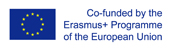This project has been funded with support from the European Commission.
This web site reflects the views only of the author, and the Commission cannot be held responsible for any use which may be made of the information contained therein.

Select language   >   IT EN RO PL LT

### Exponential Growth

Subject taught
- Math

Language Skills Developed
- Interaction
- Listening
- Speaking
- Writing

Transferable/Scientific Skills Developed
Students will develop their problem-solving skills connected with exponential growth and understand what exponential growth is and how to use it.

Description
• General aims - To be able to calculate exponential variation (degree).
To be able to draw a graph of exponential function in a given interval.
To introduce key vocabulary in English and understand the content.
• Linguistic aims – Students should be able to understand a scientific article or video.
Students should be able to present information in English using key vocabulary.
• Subject specific aims – to calculate the numerical values of the functions and draw the graphs.
• Target group age- 16/18 years old
• Level of competence in English (CEFR) – B1/B2
• Different phases to complete the lesson plan-
First step, teachers need to select key vocabulary items that learners need to know and understand in order to be able to process the input in the videos and tasks. Next, teachers introduce the degree, the basis of the degree, and the degree indicator. The possibilities of applying exponential variation in the calculation of degrees are defined and showed on the board.
Later, teachers use the video and asks students to write down key information. The video could later be discussed with students in English.
After the video, teachers put students in pairs and ask them to calculate the numerical values of the function
f (x) = 2x, where x belongs to [0; 20], using an app Function Graph Plotter. Upon completion of the activity, students present their findings in English and discuss it with the teachers.
As homework teachers can ask students to watch the video again and do some more exercises on the website and app.

• Possible difficulties for the students - Students incorrectly calculate the degree while performing mathematical operations.

Teaching Resources (created)

Teaching Resources (reviewed)